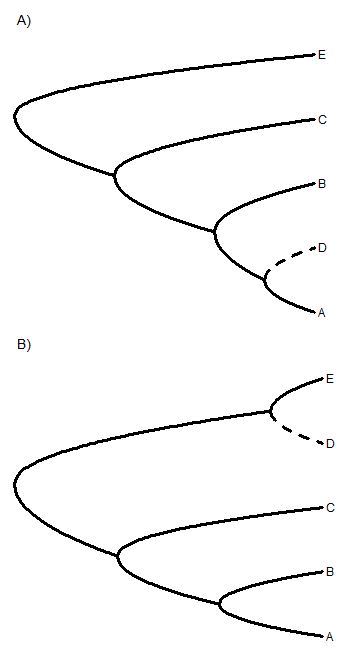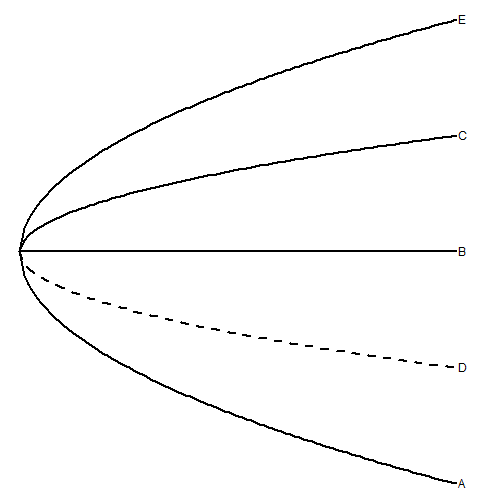## Saturday, June 11, 2016

### Update to `roundPhylogram` to permit different `lwd` and `lty` for different edges

I just pushed a small update to `roundPhylogram` that permits different line types (`lty`) and line widths (`lwd`) to be specified for different branches of the tree. This will be done whenever either argument is supplied as a vector instead of as a single scalar value or (in the case of `lty`) as a character string.

I did this so that I could plot taxa with ambiguous phylogenetic placement (say) using a dotted line. Note that it would also be straightforward to do this in functions using `plotSimmap` or the internal function `phylogram`, but this does not yet exist. It may exist in the generic S3 `plot` method for objects of class `"phylo"`. I don't know, but I wouldn't be surprised.

Here is what I mean.

``````library(phytools)
packageVersion("phytools") ## latest GitHub version
``````
``````##  '0.5.37'
``````
``````## read trees
t1<-"((((A:0.5,D:0.5):0.5,B:1):1,C:2):1,E:3);"
t2<-"(((A:1,B:1):1,C:2):1,(D:0.5,E:0.5):2.5);"

par(mfrow=c(2,1))

lty<-rep("solid",nrow(t1\$edge))
lty[which(t1\$edge[,2]==which(t1\$tip.label=="D"))]<-
"dashed"
roundPhylogram(t1,mar=c(0.1,0.1,3.1,0.1),
lty=lty,lwd=3)
lty<-rep("solid",nrow(t2\$edge))
lty[which(t2\$edge[,2]==which(t2\$tip.label=="D"))]<-
"dashed"
roundPhylogram(t2,mar=c(0.1,0.1,3.1,0.1),
lty=lty,lwd=3)
``````Note that the consensus of these two trees (similar as they are in so many respects, except with respect to the placement of `"D"`), is a star tree:

``````cons<-consensus(c(t1,t2))
lty<-rep("solid",nrow(cons\$edge))
lty[which(cons\$edge[,2]==which(cons\$tip.label=="D"))]<-
"dashed"
roundPhylogram(cons,lty=lty)
``````